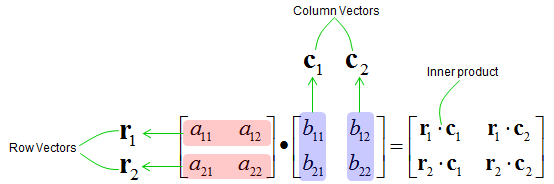Engineering Math - Quick Reference                                 Home : www.sharetechnote.com Matrix - Multiplication   How do we do multiply two matrix ? For simplicity, let's think of the multiplication of two 2x2 matrix as shown below.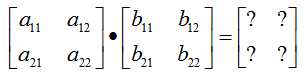Just jumping to the conclusion. The result is as shown below.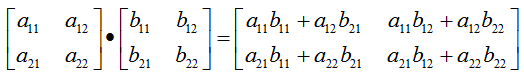How did we get this result ? What is the meaning of each elements in the resulting matrix ? Following shows you how each of the elements in resulting matrix are calculated.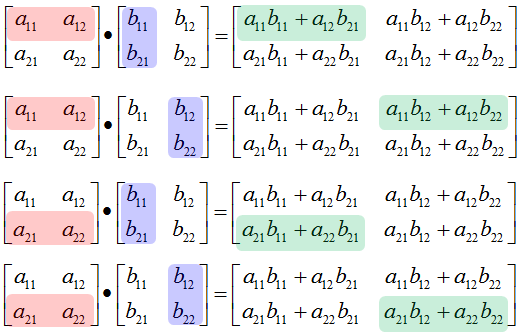If it is not clear to you yet on how each of the elements in the resulting matrix came, take a look at the following arrows. I hope it got clearer to you.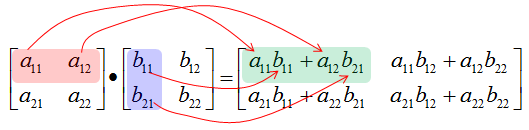If you are familiar with the concept of the inner product of two vectors, each element of the resulting matrix can be described by the inner product of a row vector in the first matrix and the column vector of the second matrix as shown below.